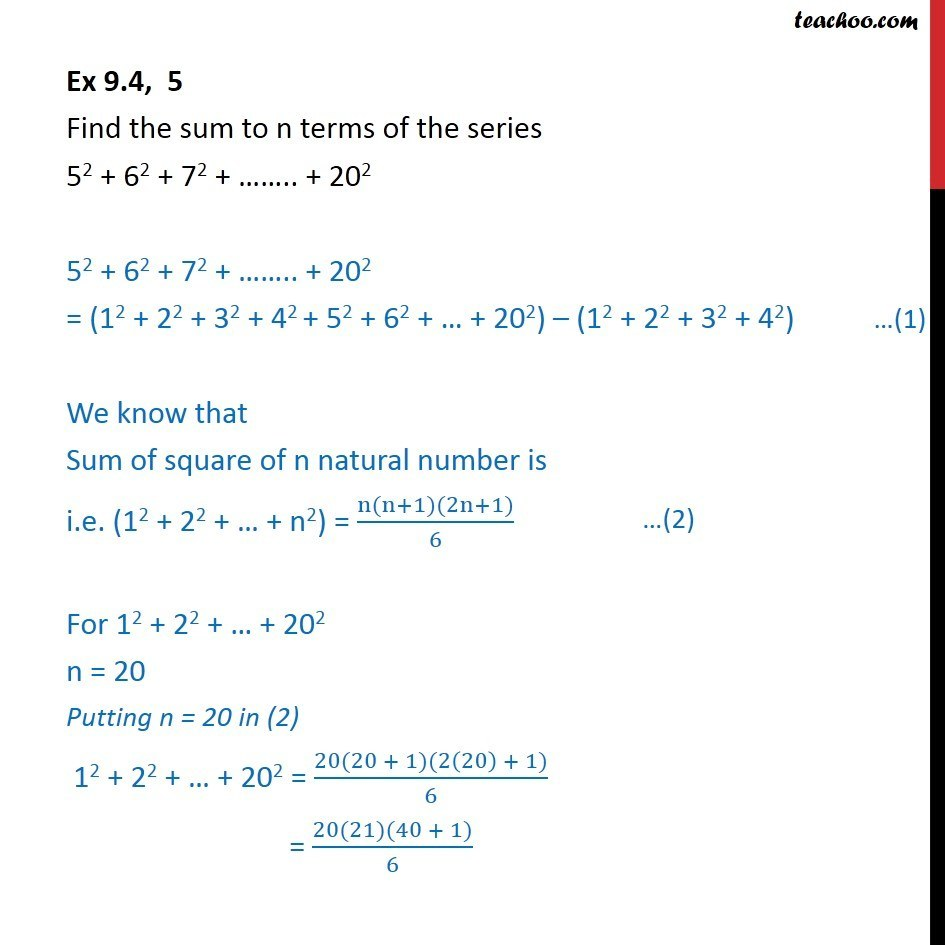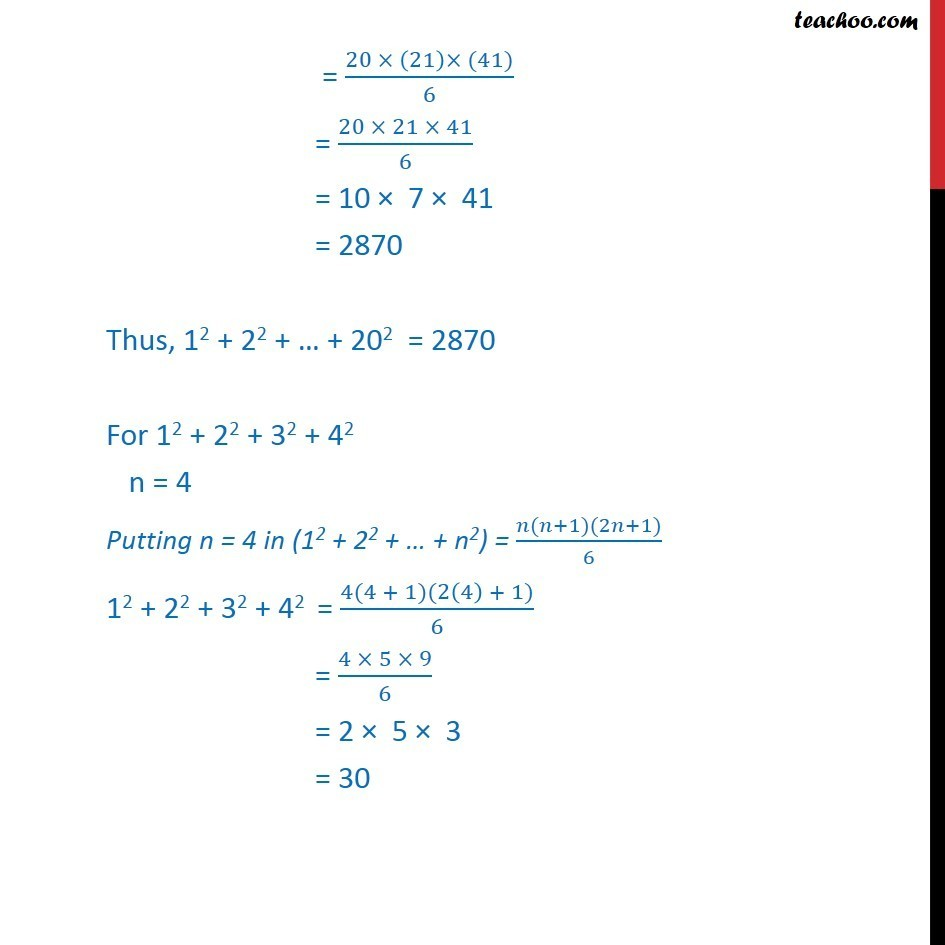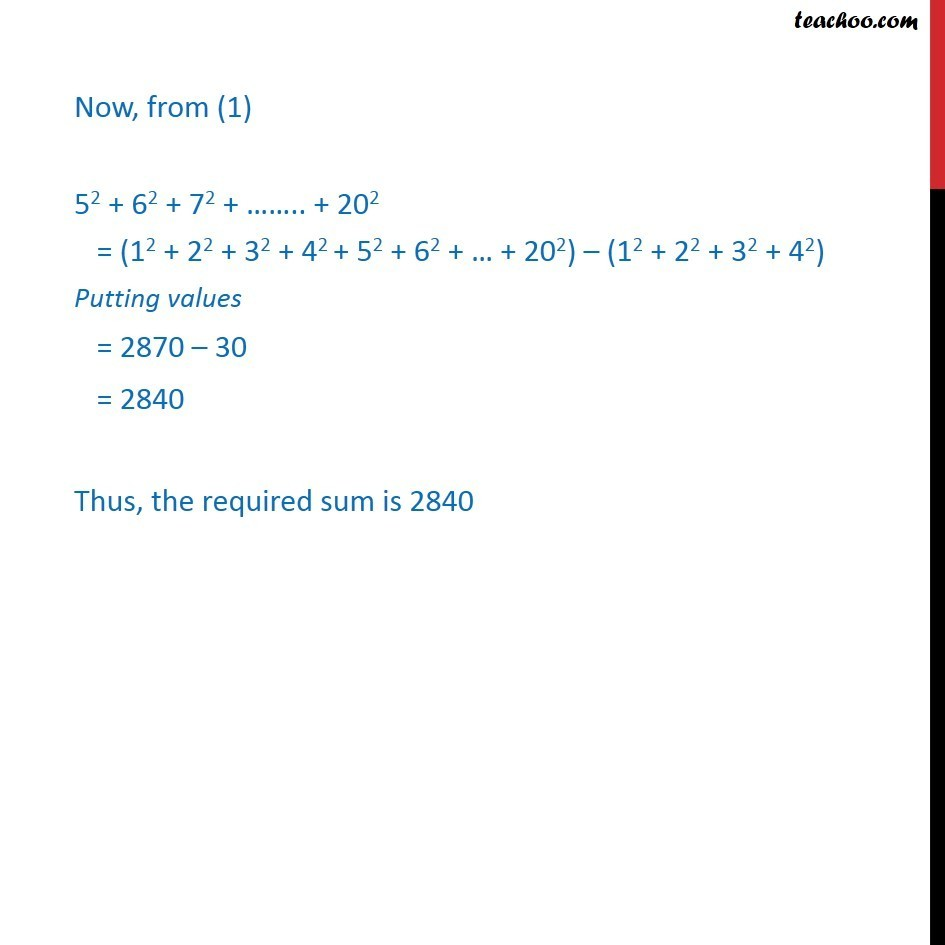Finding sum from nth number

Chapter 8 Class 11 Sequences and Series
Concept wiseLearn in your speed, with individual attention - Teachoo Maths 1-on-1 Class

### Transcript

Question 5 Find the sum to n terms of the series 52 + 62 + 72 + .. + 202 52 + 62 + 72 + .. + 202 = (12 + 22 + 32 + 42 + 52 + 62 + + 202) (12 + 22 + 32 + 42) We know that Sum of square of n natural number is i.e. (12 + 22 + + n2) = (n(n+1)(2n+1))/6 For 12 + 22 + + 202 n = 20 Putting n = 20 in (2) 12 + 22 + + 202 = (20(20 + 1)(2(20) + 1))/6 = (20(21)(40 + 1))/6 = (20 (21) (41))/6 = (20 21 41)/6 = 10 7 41 = 2870 Thus, 12 + 22 + + 202 = 2870 For 12 + 22 + 32 + 42 n = 4 Putting n = 4 in (12 + 22 + + n2) = ( ( +1)(2 +1))/6 12 + 22 + 32 + 42 = (4(4 + 1)(2(4) + 1))/6 = (4 5 9)/6 = 2 5 3 = 30 Now, from (1) 52 + 62 + 72 + .. + 202 = (12 + 22 + 32 + 42 + 52 + 62 + + 202) (12 + 22 + 32 + 42) Putting values = 2870 30 = 2840 Thus, the required sum is 2840